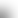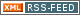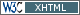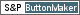www.LinuxHowtos.org howtos, tips&tricks and tutorials for linuxfrom small one page howto to huge articles all in one place

search text in:

Poll
Which filesystem do you use?

poll results

using iotop to find disk usage hogs

using iotop to find disk usage hogs

words:

887

views:

170093

userrating:

average rating: 1.7 (102 votes) (1=very good 6=terrible)

May 25th. 2007:
Words

486

Views

249283

Workaround and fixes for the current Core Dump Handling vulnerability affected kernels

Workaround and fixes for the current Core Dump Handling vulnerability affected kernels

words:

161

views:

135608

userrating:

average rating: 1.4 (41 votes) (1=very good 6=terrible)

April, 26th. 2006:

You are here: manpages

# POW

Section: Linux Programmer's Manual (3)
Updated: 2017-09-15

## NAME

pow, powf, powl - power functions

## SYNOPSIS

```#include <math.h>

double pow(double x, double y);
float powf(float x, float y);
long double powl(long double x, long double y);
```

Feature Test Macro Requirements for glibc (see feature_test_macros(7)):

powf(), powl():

_ISOC99_SOURCE || _POSIX_C_SOURCE >= 200112L
|| /* Since glibc 2.19: */ _DEFAULT_SOURCE
|| /* Glibc versions <= 2.19: */ _BSD_SOURCE || _SVID_SOURCE

## DESCRIPTION

These functions return the value of x raised to the power of y.

## RETURN VALUE

On success, these functions return the value of x to the power of y.

If x is a finite value less than 0, and y is a finite noninteger, a domain error occurs, and a NaN is returned.

If the result overflows, a range error occurs, and the functions return HUGE_VAL, HUGE_VALF, or HUGE_VALL, respectively, with the mathematically correct sign.

If result underflows, and is not representable, a range error occurs, and 0.0 is returned.

Except as specified below, if x or y is a NaN, the result is a NaN.

If x is +1, the result is 1.0 (even if y is a NaN).

If y is 0, the result is 1.0 (even if x is a NaN).

If x is +0 (-0), and y is an odd integer greater than 0, the result is +0 (-0).

If x is 0, and y greater than 0 and not an odd integer, the result is +0.

If x is -1, and y is positive infinity or negative infinity, the result is 1.0.

If the absolute value of x is less than 1, and y is negative infinity, the result is positive infinity.

If the absolute value of x is greater than 1, and y is negative infinity, the result is +0.

If the absolute value of x is less than 1, and y is positive infinity, the result is +0.

If the absolute value of x is greater than 1, and y is positive infinity, the result is positive infinity.

If x is negative infinity, and y is an odd integer less than 0, the result is -0.

If x is negative infinity, and y less than 0 and not an odd integer, the result is +0.

If x is negative infinity, and y is an odd integer greater than 0, the result is negative infinity.

If x is negative infinity, and y greater than 0 and not an odd integer, the result is positive infinity.

If x is positive infinity, and y less than 0, the result is +0.

If x is positive infinity, and y greater than 0, the result is positive infinity.

If x is +0 or -0, and y is an odd integer less than 0, a pole error occurs and HUGE_VAL, HUGE_VALF, or HUGE_VALL, is returned, with the same sign as x.

If x is +0 or -0, and y is less than 0 and not an odd integer, a pole error occurs and +HUGE_VAL, +HUGE_VALF, or +HUGE_VALL, is returned.

## ERRORS

See math_error(7) for information on how to determine whether an error has occurred when calling these functions.

The following errors can occur:

Domain error: x is negative, and y is a finite noninteger
errno is set to EDOM. An invalid floating-point exception (FE_INVALID) is raised.
Pole error: x is zero, and y is negative
errno is set to ERANGE (but see BUGS). A divide-by-zero floating-point exception (FE_DIVBYZERO) is raised.
Range error: the result overflows
errno is set to ERANGE. An overflow floating-point exception (FE_OVERFLOW) is raised.
Range error: the result underflows
errno is set to ERANGE. An underflow floating-point exception (FE_UNDERFLOW) is raised.

## ATTRIBUTES

For an explanation of the terms used in this section, see attributes(7).
 Interface Attribute Value pow(), powf(), powl() Thread safety MT-Safe

## CONFORMING TO

C99, POSIX.1-2001, POSIX.1-2008.

The variant returning double also conforms to SVr4, 4.3BSD, C89.

## BUGS

On 64-bits, pow() may be more than 10,000 times slower for some (rare) inputs than for other nearby inputs. This affects only pow(), and not powf() nor powl().

In glibc 2.9 and earlier, when a pole error occurs, errno is set to EDOM instead of the POSIX-mandated ERANGE. Since version 2.10, glibc does the right thing.

If x is negative, then large negative or positive y values yield a NaN as the function result, with errno set to EDOM, and an invalid (FE_INVALID) floating-point exception. For example, with pow(), one sees this behavior when the absolute value of y is greater than about 9.223373e18.

In version 2.3.2 and earlier, when an overflow or underflow error occurs, glibc's pow() generates a bogus invalid floating-point exception (FE_INVALID) in addition to the overflow or underflow exception.

cbrt(3), cpow(3), sqrt(3)

## Index

NAME
SYNOPSIS
DESCRIPTION
RETURN VALUE
ERRORS
ATTRIBUTES
CONFORMING TO
BUGS
COLOPHON||- Powered by- Running on-
Copyright 2004-2020 Sascha Nitsch Unternehmensberatung GmbH::- Level Triple-A Conformance to Web Content Accessibility Guidelines 1.0 -
- Copyright and legal notices -